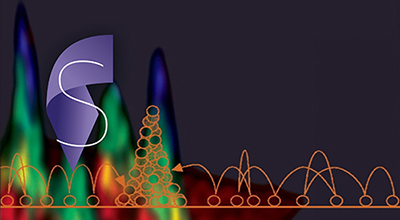Physics
##### We explore statistical physics in both classical and open quantum systems. Additionally, we will cover probabilistic data analysis that is extremely useful in many applications.Course Details

 Language English Duration 10 weeks Effort 3 to 5 hours a week
##### Description

This course covers non-equilibrium statistical processes and the treatment of fluctuation dissipation relations by Einstein, Boltzmann and Kubo. Moreover, the fundamentals of Markov processes, stochastic differential and Fokker Planck equations, mesoscopic master equation, etc will be treated in detail. Prior knowledge of statistical physics is highly recommended but not required.

##### What you will learn

• Formulate statistical processes mathematically

• Solve the quantum master equation using QuTip in Python

• Apply numerical simulation tools to non-equilibrium systems

• Explore the quantum optical numerical Toolbox (MATLAB)

• Visualize non-equilibrium processes numerically using Jupyter Notebooks

• Elaborate modern examples from Literature of Non-Equilibrium Processes

• Apply EMCEE Python package to Bayesian statistical data analysis

##### Prerequisites

Graduate level knowledge of mathematics and physics

##### Plan

Lecture 1: Brownian motion and 3 derivations

Lecture 2: Continuous stochastic process

Lecture 3: Stochastic differential equations

Lecture 4: Fluctuation dissipation theorem

Lecture 5: Fokker Planck equation

Lecture 6: Lévy flights

Lecture 7: Master equations

Lecture 8: The Crook and Jarzynski equality

Lecture 9+10: Quantum optics and quantum Langevin equation

Lecture 11+12: Quantum regression theorem

### Course instructors

##### Tobias J. Kippenberg

Tobias J. Kippenberg is Full Professor of Physics at EPFL and leads the Laboratory of Photonics and Quantum Measurement. He obtained his BA at the RWTH Aachen, and MA and PhD at the California Institute of Technology (Caltech in Pasadena, USA), in 1998, 2…

##### École polytechnique fédérale de Lausanne

Free online courses from École polytechnique fédérale de Lausanne

EPFL is the Swiss Federal Institute of Technology in Lausanne. The past decade has seen EPFL ascend to the very top of European institutions of science and technology: it is ranked #1 in E…

93 instructors